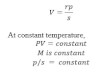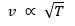# Physical Factors Affecting Of sound

1. Effect of pressure (at constant temp)Thus, velocity of sound is independent of change of pressure at given temperature.

2. Effects of temperature
Since the velocity of sound in gas,i.e. the velocity of sound in gas or air is directly proportional to the square root of absolute temperature.

3. Effect of density
At constant pressure,
Velocity of sound in gas is inversely prop to sq. root of density.

4. Effect of humidity
The of water vapor is small that of dry air i.e. presence of moisture in air reduce density of air, since the velocity of sound in gas is inversely proportional to sq. root of its density then velocity of sound in humid air is greater than that of dry air.

5. Effect of wind
If the air is flowing, the speed of the sound changes. If wind blow in direction of sound, velocity of sound increases and vice versa.

6. Effect of frequency wave length & amplitude
Velocity of sound in air is independent of frequency wave length. Sound wave of different frequencies travel with the velocity in air though their wavelength in air are different the velocity is also independent of amplitude as well however if amplitude is very large, the compression and rarefaction may result in large temperature variation and this affect the velocity of sound.